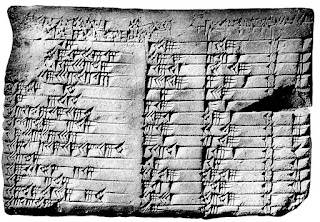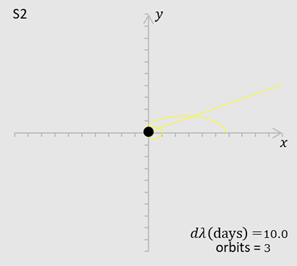## Saturday, 28 November 2020

### Diagonal metric and Ricci tensorGregorio Ricci-Curbastro
Just as I was wrapping up section 8.2 on the Robertson-Walker I wondered if the Ricci tensor was always diagonal if the metric was diagonal. The R-W metric and Ricci tensor are both diagonal and Carroll had not said anything about off diagonal components of the Ricci tensor except that they vanished. I was dissatisfied and about to ask on Physics Forums. Then I decided to try to check myself, fumbled for a bit and then found a 1996 paper by K.Z. Win. I suppose in this day and age of Mathematica and the like it has sunk into obscurity but nevertheless it was interesting and useful for me who lacks such tools.

Win shows that for a diagonal metric the diagonal components of the Ricci tensor are$$4R_{\mu\mu}=\left(\partial_\mu\ln{\left|g_{\mu\mu}\right|}-2\partial_\mu\right)\partial_\mu\ln{\left|\frac{g}{g_{\mu\mu}}\right|}-\sum_{\sigma\neq\mu}\left[\left(\partial_\mu\ln{\left|g_{\sigma\sigma}\right|}\right)^2+\left(\partial_\sigma\ln{\frac{\left|g\right|}{{g_{\mu\mu}}^2}}+2\partial_\sigma\right)g^{\sigma\sigma}\partial_\sigma g_{\mu\mu}\right]$$and the off diagonal components are $$4R_{\mu\nu}=\left(\partial_\mu\ln{\left|g_{\nu\nu}\right|}-\partial_\mu\right)\partial_\nu\ln{\left|\frac{g}{g_{\mu\mu}g_{\nu\nu}}\right|}+\left(\mu\leftrightarrow\nu\right)-\sum_{\sigma\neq\mu,\nu}{\partial_\mu\ln{\left|g_{\sigma\sigma}\right|}\partial_\nu\ln{\left|g_{\sigma\sigma}\right|}}$$where $\mu\neq\nu$, there is no summation over $\mu,\nu$ and $\left(\mu\leftrightarrow\nu\right)$  stands for preceding terms with $\mu,\nu$ interchanged. The partial derivative operator $\partial_\tau$ usually only applies to what immediately follow. $\partial_\tau\partial_\tau$ is a second order derivative. The exception is the final $2\partial_\sigma$ in the first expression which applies to the whole of $g^{\sigma\sigma}\partial_\sigma g_{\mu\mu}$.

It turns out that in two dimensions a diagonal metric does imply a diagonal Ricci tensor - but we knew that. In more dimensions it's not so simple. It is necessary to calculate the off diagonal components of the R-W Ricci tensor.

To calculate the Ricci tensor more directly one would use $$R_{\mu\mu}=R_{\ \ \mu\sigma\mu}^\sigma=\partial_\sigma\Gamma_{\mu\mu}^\sigma-\partial_\mu\Gamma_{\sigma\mu}^\sigma+\Gamma_{\sigma\rho}^\sigma\Gamma_{\mu\mu}^\rho-\Gamma_{\mu\rho}^\sigma\Gamma_{\sigma\mu}^\rho$$ and$$R_{\mu\nu}=R_{\ \ \mu\sigma\nu}^\sigma=\partial_\sigma\Gamma_{\nu\mu}^\sigma-\partial_\nu\Gamma_{\sigma\mu}^\sigma+\Gamma_{\sigma\rho}^\sigma\Gamma_{\nu\mu}^\rho-\Gamma_{\nu\rho}^\sigma\Gamma_{\sigma\mu}^\rho$$and once the Christoffel symbols have been calculated, which is quite easy in the R-W case,  the former takes about the same number of lines as Win's formula but the latter is probably much less efficient than Win. However Win's method avoids calculating Christoffel symbols at all.

Read the full details at Commentary 8.2 Diagonal metric and Ricci tensor.pdf (11 pages)

## Tuesday, 17 November 2020

### Constants and calculationsBabylonian equations
Carroll uses natural units in his book as do I on this web site. In natural units the speed of light, Planck's constant and Boltzmann's constants $c=\hbar=h/2\pi=k=1$. In our equations these terms are left out. They need to be put in again to do real calculations and it's not always obvious how to do it. When Carroll does calculations he often uses centimeters and grams. I prefer to use SI units: meters and kilograms.

It is also common to use geometric units which are units in which $c=G=1$ (so mass and energy have the same units as length and time). You cannot also have $\hbar=1$ in these units.

In natural units the Schwarzschild radius is $R_S=2GM$. I suppose that in geometric units it is $R_S=2M$. In SI units it is $2GM/c^2$. The $c^2$ makes a big difference and is easy to forget!

The document contains a list in SI units of the values of universal constants and other handy constants like the mass of the sun. The table also gives the conversion factors from natural to SI units, which are derived from their dimensions. Two examples of their use are given at the end followed by a list of small and large prefixes (Terra, peta, pico etc) and their meanings.

## Tuesday, 10 November 2020

### Plotting geodesics of Schwarzschild

I did some experiments with trying to plot solutions to the geodesics of Schwarzschild. They might give orbits of stars round the black hole Sagittarius A* at the centre of our galaxy. The geodesic equations become three simultaneous second order differential equations which give what I call the chugger equations below:$t$ is the coordinate time and $r,\theta$ are the usual plane polar coordinates. We are only considering curves in one plane. A prime indicates a derivative with respect to $\lambda$ which is the affine parameter from the geodesic equation and could be proper time. $G$ is old Newton's gravitational constant, $M$ is the mass of Sagittarius A* and $c$ is the speed of light.We start with some initial values of $t,t^\prime,r,r^\prime,\theta,\theta^\prime$ and select some $d\lambda$ . Then we use those to get new values of  $t,t^\prime,r,r^\prime,\theta,\theta^\prime$ from the chugger equations again and again and again and put them in successive rows of a spreadsheet. The spreadsheet then uses the values of $r,\theta$ to plot the curve. One of the stars orbiting Sagittarius A* is S2 and we use that as our example. It completes an orbit about every 16 years. The first image was my first attempt. The shape is good (it follows the top part of the Newtonian ellipse) until it gets pretty close to the black hole. The second image shows a close up of the same curve near the black hole.

The dashed line is the ellipse that S2 would follow according to Newton's equations.

If I 'manually' decreased $d\lambda$ when near the black hole and then increased it again as S2 departed I could get the third image which shows the last leg of the approximation. To be able to do that conveniently I had to program the chugger equations in VBA. To do 1,000 iterations takes about three minutes.

Finally I did all the calculations in Excel adjusting $d\lambda$ as it went along and got the fourth image from a 20,000 row spreadsheet. The calculation time is about one second. It was the best so far but still not good enough. The furthest distance (apsis) of S2 from the black hole decreases by 4% - it should be the same. However the furthest distance did advance by 0.0012 radians. I calculated (with help from Carroll) that it should be 0.0035 radians.

For a bit of fun I also made an artist's impression of precession of the perihelion. It's simply done from the exact solution to Newton's equations which is an ellipse.

Further material including spreadsheets and VBA at

## Tuesday, 3 November 2020

### Solar orbit plotter

Here's how the plotter works
Newton's second law is$$\vec{F}=m\vec{a}$$Newton's law of gravity is$$\vec{F}=\frac{GMm}{r^2}$$They give you two differential equations of motion in polar coordinates$$\frac{d^2r}{dt^2}-r\left(\frac{d\theta}{dt}\right)^2=-\frac{GM}{r^2}$$$$2\frac{dr}{dt}\frac{d\theta}{dt}+r\frac{d^2\theta}{dt^2}=0$$Kepler's laws are
1. The orbit of a planet is an ellipse with the Sun at one of the two foci.
2. A line segment joining a planet and the Sun sweeps out equal areas during equal intervals of time.
3. The square of a planet's orbital period is proportional to the cube of the length of the semi-major axis of its orbit.
The second differential equation quickly gives you Kepler's second law. It is more difficult to get$$r=\frac{P}{1+e\cos{\left(\theta-\phi\right)}}$$That is a circle when $e=0$, an ellipse when $\left|e\right|<1$, a parabola when $\left|e\right|=1$ and a hyperbola when $\left|e\right|>1$. $\phi$ is the angle of the axis of symmetry of the curve. $P$ is a magic constant. The equation gives you Kepler's first law.

Newton must have been very pleased when he did those. Part of the job was inventing calculus!

The initial conditions determine $P,e,\phi$. That requires more work if you only know the initial position and velocity.

Full details (except inventing calculus) in Commentary 5.4 Orbital toypdf. (9 pages)
The spreadsheets for plotting the curves are at
Commentary 5.4 Orbital toy.xlsm (with animation macros)